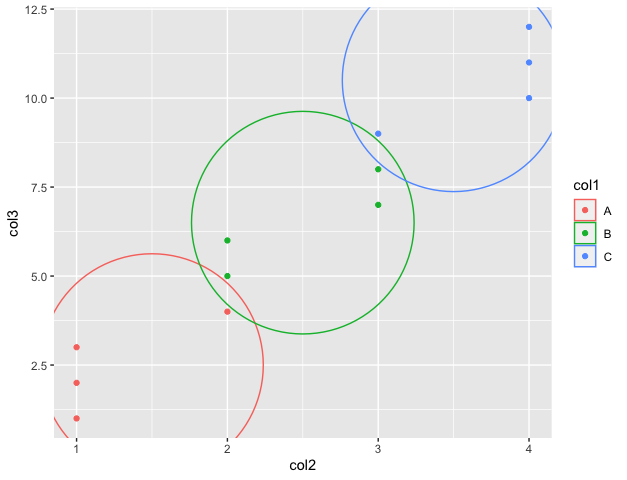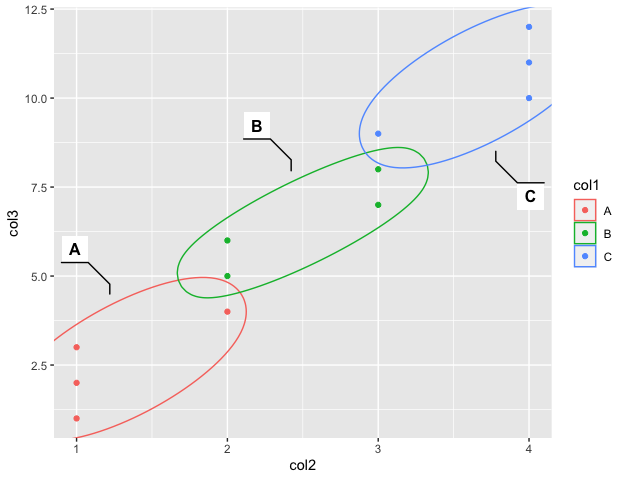# How to Annotate a Specific Cluster or Group in ggplot2 in R?

• Last Updated : 28 Nov, 2021

The ggplot method in R Programming Language is used to do graph visualizations using the specified dataframe. It is used to instantiate a ggplot object. Aesthetic mappings can be created to the plot object to determine the relationship between the x and y-axis respectively. Additional components can be added to the created ggplot object.

Syntax: ggplot(data = NULL, mapping = aes(), fill = )

Arguments :

data – Default dataset to use for plot.

mapping – List of aesthetic mappings to use for plot.

Geoms can be added to the plot using various methods. The geom_point() method in R can be used to add points to the scatter plot in the graph created in R, which may or may not contain aesthetic mappings, for instance, color.

`geom_point(aes(color= ))`

Color can be assigned to the respective groups of the column of the data frame. The points in the data frame can be labeled using dots in the graph. A scatter plot can therefore be created by creating the points. These points may or may not belong to the same groups. These groups can be labeled differently in the graph.

## Method 1: Using geom_mark_circle package

The geom_mark_circle geom method allows the user to annotate sets of points via circles. The method can contain a set of aesthetic mappings, which are specified using color, position, or labels.

`geom_mark_circle(aes(color = ))`

The points can be assigned different colors based on the grouping column value to which they correspond. These points are marked using different colors. Then the circles are constructed around them using the geom_mark_circle() method in R. This segregates the points marked belonging to different groups. Initially, the col1 points are marked as x ax-axisxis points and col2 as y-axis points corresponding to the data frame. The points are marked belonging to different colors which can be specified using the aesthetic mappings and added as components to the ggplot() method. The points are assigned colors using the geom_point() component.

## R

 `# importing the required library ` `library``(ggplot2) ` `data_frame < - ``data.frame``(stringsAsFactors=``FALSE``, ` `                          ``col1=``c``(``rep``(``LETTERS``[1:3], each=4)), ` `                          ``col2=``c``(``rep``(1: 4, each=3)), ` `                          ``col3=``c``(1: 12)) ` `print``(``"original dataframe"``) ` `print``(data_frame) ` `data_frame % >% ` `ggplot``(``aes``(x=col2, ` `           ``y=col3))+ ` ` `  `# marking circles ` `geom_mark_circle``(``aes``(color=col1))+ ` ` `  `# marking points on the plot ` `geom_point``(``aes``(color=col1)) `

Output

``` "original dataframe"
> print(data_frame)
col1 col2 col3
1     A    1    1
2     A    1    2
3     A    1    3
4     A    2    4
5     B    2    5
6     B    2    6
7     B    3    7
8     B    3    8
9     C    3    9
10    C    4   10
11    C    4   11
12    C    4   12```## Method 2: Using geom_mark_ellipse method

The geom_mark_ellipse() geom method allows the user to annotate sets of points via circles. The method can contain a set of aesthetic mappings, which are specified using color, position. Labels can be assigned too using the label argument in this method.

`geom_mark_circle(aes(color = , label =  ))`

The points can be assigned different colors based on the grouping column value to which they correspond. These points are marked using different colors. Then the ellipses are constructed around them using the geom_mark_ellipse() method in R. This segregates the points marked belonging to different groups.

## R

 `# importing the required library ` `library``(tidyverse) ` `data_frame < - ``data.frame``(stringsAsFactors=``FALSE``, ` `                          ``col1=``c``(``rep``(``LETTERS``[1:3], each=4)), ` `                          ``col2=``c``(``rep``(1: 4, each=3)), ` `                          ``col3=``c``(1: 12)) ` `print``(``"original dataframe"``) ` `print``(data_frame) ` `data_frame % >% ` `ggplot``(``aes``(x=col2, ` `           ``y=col3))+ ` ` `  `geom_mark_ellipse``(``aes``(color=col1, ` `                      ``label=col1), ` `                  ``)+ ` ` `  `geom_point``(``aes``(color=col1)) `

Output

``` "original dataframe"
> print(data_frame)
col1 col2 col3
1     A    1    1
2     A    1    2
3     A    1    3
4     A    2    4
5     B    2    5
6     B    2    6
7     B    3    7
8     B    3    8
9     C    3    9
10    C    4   10
11    C    4   11
12    C    4   12```My Personal Notes arrow_drop_up
Recommended Articles
Page :